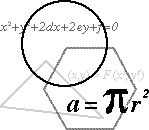EXPRESIONES MATEMATICAS Mathematical expressionsLOS CURSOS DE INGLES GRATIS PREFERIDOS POR LOS HISPANOHABLANTES

 Audio

 + plus Some examples - minus 6 + 9 = 15 Six plus nine equals / is fifteen. X times, multiplied by 5 x 6 = 30 Five times six equals thirty or : divided by Five multiplied by six is thirty. = equals 75% Seventy-five per cent of the % per cent class passed the test. 32 three squared 53 five cubed610 six to the power of ten (UK) six to the tenth power (US)